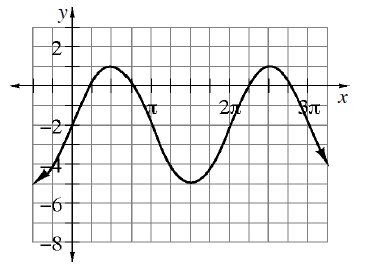### Home > PC3 > Chapter 4 > Lesson 4.3.1 > Problem4-116

4-116.This problem is a checkpoint for writing equations of and graphing trigonometric functions.

1. Write an equation for the graph below.2. Graph two cycles of $y=-2\cos(x)+1$.

3. Write the equation of your graph from part (b) as a transformation of the sine function.